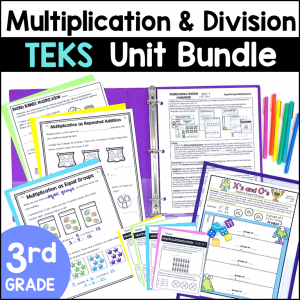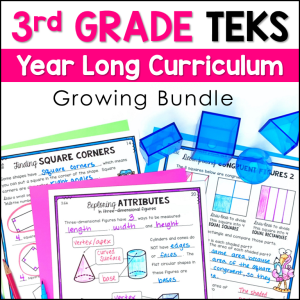Save 10% on your first order with code EXTRA10

# 3rd Grade Multiplication Division Set 1 – STAAR Review Task Cards – Digital

\$4.00\$29.99\$151.25\$23.50
SKU: 5578302 Categories: ,

## Description

This resource includes 24 TEKS-aligned Digital Task Cards for Multiplication and Divsion. There are a variety of problems with arrays, repeated addition, number lines, missing factors or products, finding odd and even numbers and solving word problems. This resource can be assigned for Distance Learning via Google Classroom. These task cards are in a Google Form, which means they are automatically graded when students click submit!

The questions are rigorous and have bright graphics to keep students engaged. All questions are multiple choice and are STAAR formatted.

I have divided the Multiplication and Division Review into 2 sets. This resource is Multiplication and Division Review Set #1. Set 1 covers the basics of multiplication and division and includes all supporting standards. Set 2 will cover moved advanced topics such as multi-step word problems, 2×1 multiplication, and tables to represent real-word relationships.

This resource can be used as a 24 question review activity, but it can also be used in many other ways! You can make a copy of the Google Form and delete questions to differentiate. There are 24 questions included, so you also have the ability to create multiple reviews from this resource, splitting up the questions to use in a variety of ways. Please note that the questions are not editable, but most of the answer choices are editable.

You do not need Google Classroom to use this resource, but you must have a Google e-mail to use Google Forms.

What’s Included?

• Printable Teacher Tracking sheet to record names of students who are struggling with each of the TEKS (based on the problem numbers they missed)

Topics include:

• One-step word problems
• Arrays
• Representing Multiplication with Repeated Addition, Skip Counting, Jumps on a Number Line and arrays
• Using Multiplication facts to solve Division problems
• Division of objects into Equal Groups, or taking out Equal Shares
• Odd and Even Numbers
• Describing Multiplication as a Comparison
• Finding Missing Factors and Products in Multiplication and Division equations

TEKS Alignment:

Supporting Standards

3.4D: (3 questions) Determine the total number of objects when equally sized groups of objects are combined or arranged in arrays up to 10 by 10.

3.4E: (3 questions) Represent multiplication facts by using a variety of approaches such as repeated addition, equal-sized groups, arrays, area models, equal jumps on a number line, and skip counting.

3.4F: (3 questions) Recall facts to multiply up to 10 by 10 with automaticity and recall the corresponding division facts.

3.4H: (3 questions) Determine the number of objects in each group when a set of objects is partitioned into equal shares or a set of objects is shared equally.

3.4I: (3 questions) Determine if a number is even or odd using divisibility rules.

3.4J: (3 questions) Determine a quotient using the relationship between multiplication and division.

3.5C: (3 questions) Describe a multiplication expression as a comparison such as 3 x 24 represents 3 times as much as 24.

3.5D: (3 questions) Determine the unknown whole number in a multiplication or division equation relating three whole numbers when the unknown is either a missing factor or product.